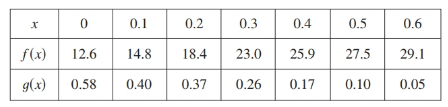# Find the value of h ' ( 0.5 ) using given relation and table.### Single Variable Calculus: Concepts...

4th Edition
James Stewart
Publisher: Cengage Learning
ISBN: 9781337687805### Single Variable Calculus: Concepts...

4th Edition
James Stewart
Publisher: Cengage Learning
ISBN: 9781337687805

#### Solutions

Chapter 3.4, Problem 57E
To determine

## Find the value of h'(0.5) using given relation and table.

Expert Solution

The value of the given expression h'(0.5)=17.4 .

### Explanation of Solution

Given:

The given relation is h(x)=f(g(x)) and the table is given below.Calculation:

Use formula h'(x)=(f(g(x)))'=f'(g(x))g'(x)

g(0.5)=0.1g'(0.5)=0.050.170.60.4=0.120.2=0.6f'(g(0.5))=f'(0.1)=18.412.60.20=5.80.2=29

Now

h'(0.5)=(f(g(0.5)))'=f'(g(0.5))g'(0.5)h'(0.5)=(f(g(0.5)))'=29(0.6)=17.4

Hence thevalue of the given expression h'(0.5)=17.4 .

### Have a homework question?

Subscribe to bartleby learn! Ask subject matter experts 30 homework questions each month. Plus, you’ll have access to millions of step-by-step textbook answers!